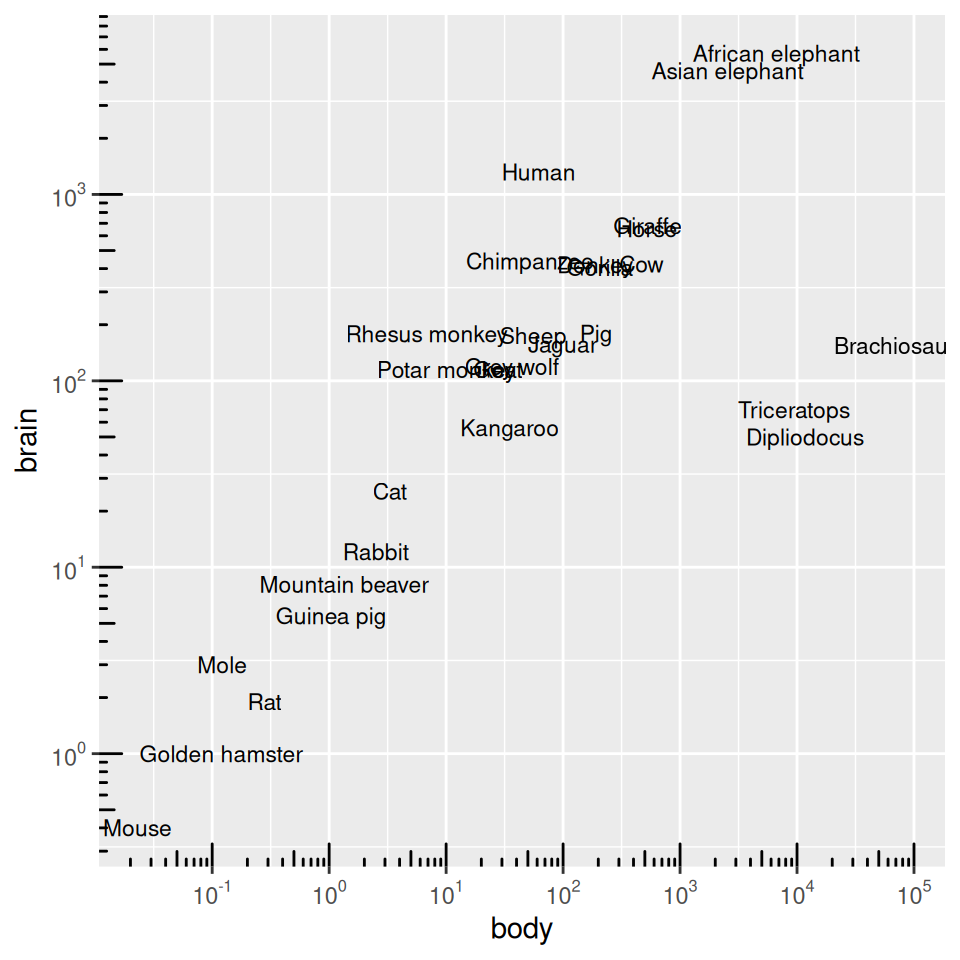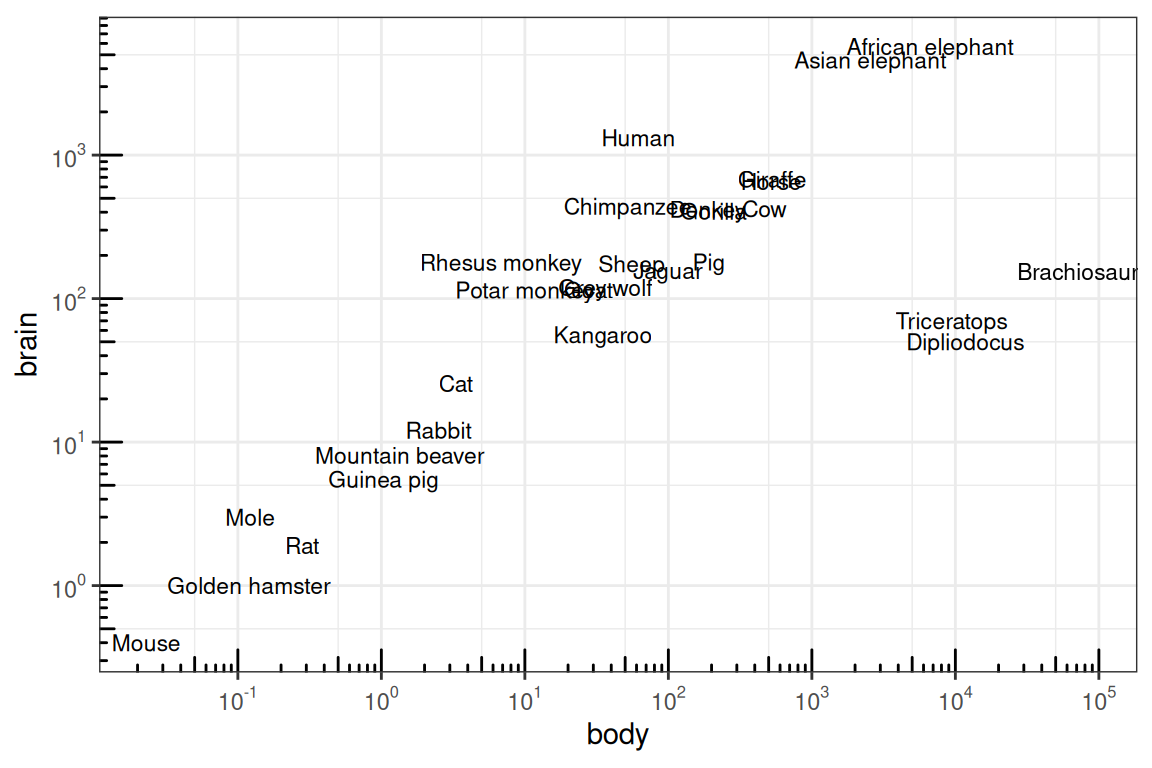## 8.15 Adding Ticks for a Logarithmic Axis

### 8.15.1 Problem

You want to add tick marks with diminishing spacing for a logarithmic axis.

### 8.15.2 Solution

Use `annotation_logticks()` (Figure 8.31):

``````library(MASS)   # Load MASS for the Animals data set
library(scales) # For the trans_format function

# Given a vector x, return a vector of powers of 10 that encompasses all values
# in x.
breaks_log10 <- function(x) {
low <- floor(log10(min(x)))
high <- ceiling(log10(max(x)))

10^(seq.int(low, high))
}

ggplot(Animals, aes(x = body, y = brain, label = rownames(Animals))) +
geom_text(size = 3) +
annotation_logticks() +
scale_x_log10(breaks = breaks_log10,
labels = trans_format(log10, math_format(10^.x))) +
scale_y_log10(breaks = breaks_log10,
labels = trans_format(log10, math_format(10^.x)))``````Figure 8.31: Log axes with diminishing tick marks

We also defined a function, `breaks_log10()`, which returns all powers of 10 that encompass the range of values passed to it. This tells `scale_x_log10` where to put the breaks. For example:

``````breaks_log10(c(0.12, 6))
#>   0.1  1.0 10.0``````

### 8.15.3 Discussion

The tick marks created by `annotation_logticks()` are actually geoms inside the plotting area. There is a long tick mark at each power of 10, and a mid-length tick mark at each 5.

To get the colors of the tick marks and the grid lines to match up a bit better, you can use `theme_bw()`.

By default, the minor grid lines appear visually halfway between the major grid lines, but this is not the same place as the “5” tick marks on a logarithmic scale. To get them to be the same, we can supply a function for the scales `minor_breaks`.

We’ll define `breaks_5log10()`, which returns 5 times powers of 10 that encompass the values passed to it.

``````breaks_5log10 <- function(x) {
low <- floor(log10(min(x)/5))
high <- ceiling(log10(max(x)/5))

5 * 10^(seq.int(low, high))
}

breaks_5log10(c(0.12, 6))
#>   0.05  0.50  5.00 50.00``````

Then we’ll use that function for the `minor breaks` (Figure 8.32):

``````ggplot(Animals, aes(x = body, y = brain, label = rownames(Animals))) +
geom_text(size = 3) +
annotation_logticks() +
scale_x_log10(breaks = breaks_log10,
minor_breaks = breaks_5log10,
labels = trans_format(log10, math_format(10^.x))) +
scale_y_log10(breaks = breaks_log10,
minor_breaks = breaks_5log10,
labels = trans_format(log10, math_format(10^.x))) +
coord_fixed() +
theme_bw()``````Figure 8.32: Log axes with ticks at each 5, and fixed coordinate ratio